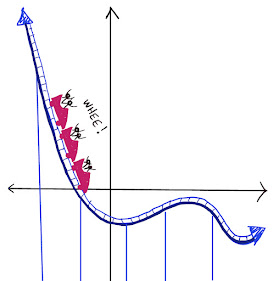## Tuesday, March 15, 2022

### Lattice Polygons, Part 2

Lattice polygons are polygons are formed by connecting dots on square dot paper.  (On a coordinate graph, the coordinates of the vertices are integers.).

The lattice polygon below is a rectangle whose dimensions are 2 units by 4 units.  Its area is 8 square units.

(1)  Can you make a small change to the rectangle so that the area of the new polygon is 6 square units? There are lots of ways to do this.

(2)  How many different kinds of polygons can you make on a geoboard with an area of exactly 6 square units?  Can you make a triangle?  a quadrilateral?  a 5-sided figure?  6-sided?  7-sided?  8-sided?

It is convenient to use this online geoboard:  Geoboard

For the solution, click below:

(1) There are lots of ways to change the figure to have an area of 6.  Here are some:

(2) The figures above are 4 and 5-sided figures.  You can make lots of different figures with almost any number of sides.  Here is a 10-sided figure with an area of 6!